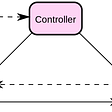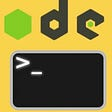`var x = 1;function f() {    let x = x;    // Something that modifies x};f();`

`let x = 3;if(x === 3) {    let y = 4;    console.log(x);    // 3    console.log(y);    // 4}console.log(x);        // 3console.log(y);        // ReferenceError`
`let x = 3;if(x === 3) {    let x = 4;    console.log(x);    // 4}console.log(x);        // 3`
• Variable shadowing is completely valid JavaScript.
• When within the scope of the local `x` (the `if` block), there is no way to access the global `x`.

# Hoisting

`function f() {    console.log(x);    // undefined    var x = 3;    console.log(x);    // 3}`
`function f() {    var x;    console.log(x);    // undefined    x = 3;    console.log(x);    // 3}`
• A variable may be defined before its first appearance in the code, possibly catching you by surprise.
• Even if you assign a variable at the same time it is declared, accessing that variable still may return `undefined`.

`function f() {    console.log(x);    // RefenceError    let x = 3;    console.log(x);}`
`function f() {    let x;// TDZ begins here    console.log(x);    // ReferenceError// TDZ ends here    x = 3;    console.log(x);}`
• If we attempt to access a `let` / `const` variable after it is defined but before it is assigned a value, we get an error.
• This differs from `var` variables, which would return `undefined` in the same situation.

# What’s wrong with my code?

`var x = 1;function f() {    let x = x;    // Something that modifies x};f();`
`var x = 1;function f() {    let x;    x = x;    // Something that modifies x};f();`

`var x = 1;function f() {    var x = x;    // Something that modifies x};f();`

# How can fix this?

`var x = 1;function f() {    let xCopy = x;    // Something that modifies xCopy};f();`

# Scope

• What is a closure?
• Exactly where does one scope end and another begin?
• Exactly what is the difference between `let`, `const` and `var`?

--

--

--

## More from Alex Bostock

https://alexbostock.co.uk

Love podcasts or audiobooks? Learn on the go with our new app.

## SAP Tutorial: Complete CAP Java 14## JavaScript Best Practices for Writing More Robust Code — Functions## Dynamic website with Django## Twitter Lite and High Performance React Progressive Web Apps at Scale## Best of Modern JavaScript — Module Details## React Hooks —  useEffect() and Its Different Use Cases — Master in 2 Minutes## Getting Started with Vue.js## JavaScript Best Practices- Modules## Alex Bostock

https://alexbostock.co.uk

## React JS vs Angular- Know ins and Outs## How to create a function that will return an array with words inside a text## Lets get user inputs using inquirer and Yargs — Creating a npm command line Utility — Part 2